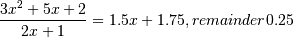# numpy.polydiv¶

numpy.polydiv(u, v)[source]

Returns the quotient and remainder of polynomial division.

The input arrays are the coefficients (including any coefficients equal to zero) of the “numerator” (dividend) and “denominator” (divisor) polynomials, respectively.

Parameters: u : array_like or poly1d Dividend polynomial’s coefficients. v : array_like or poly1d Divisor polynomial’s coefficients. q : ndarray Coefficients, including those equal to zero, of the quotient. r : ndarray Coefficients, including those equal to zero, of the remainder.

See also

Notes

Both u and v must be 0-d or 1-d (ndim = 0 or 1), but u.ndim need not equal v.ndim. In other words, all four possible combinations - u.ndim = v.ndim = 0, u.ndim = v.ndim = 1, u.ndim = 1, v.ndim = 0, and u.ndim = 0, v.ndim = 1 - work.

Examples>>> x = np.array([3.0, 5.0, 2.0])
>>> y = np.array([2.0, 1.0])
>>> np.polydiv(x, y)
(array([ 1.5 ,  1.75]), array([ 0.25]))


numpy.polyadd

numpy.polymul